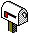# Science

• The Lonely Runner Problem
At time zero, k participants depart from the origin of a unit length circular track to run repeated laps. Each runner maintains a constant nonzero speed. Is it true that regardless of what the speeds are, there exists a time at which the k runners are simultaneously at least 1/(k+1) units from the starting point? The term "lonely runner" respects an equivalent formulation in which there are k+1 runners with distinct speeds. Is there a time at which a given runner is "lonely", that is, at distance at least 1/(k+1) from the others?
The Lonely Runner Conjecture, stated in the '60, has been proved for k
<7 in 2001. It is now a theorem since June 2016 (see here for the complete solution).

• Limits of Mathematics
One normally thinks that everything that is true is true for a reason. I've found mathematical truths that are true for no reason at all. These mathematical truths are beyond the power of mathematical reasoning because they are accidental and random.

gavoille@labri.fr